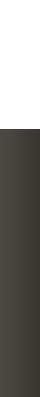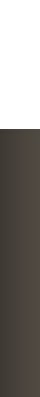Photo © Ian Coristine/1000IslandsPhotoArt.comYou are here: Search

Part 4–Sudoku Gordonian Rectangles and Polygons by Dan LeKander

 Editor’s note:  Do you tackle a Sudoku on your cottage veranda, sailboat cockpit, or at a campsite?  TI Life is taking full advantage of Dan LeKander, from Wellesley Island, who is a Sudoku expert and author of “3 Advanced Sudoku Techniques – That Will Change Your Game Forever!”  This is Part 4 of 6 Parts of his 8 Step Approach

PART 4 – GORDONIAN RECTANGLES AND POLYGONS

Recap in past issues:

STEP 3 … GORDONIAN RECTANGLES & POLYGONS

(and variations of both)

This is perhaps the most important Sudoku solving technique that has ever been published! It cuts to the heart and soul of Sudoku puzzles. One of the rules of Sudoku puzzles is there can be only one answer to a puzzle. This technique is all about ensuring that you arrive at a unique answer to a puzzle.

There is more than one name for this technique. Sudoku aficionado and puzzle book author Peter Gordon has been given credit for this technique, thus “Gordonian” has emerged. I sort of like the ring to it.

This is not a difficult technique, although some variations are a little tricky. Example 1 below illustrates the simplest essence of the technique.

Assume example 1 blank cells are solved cells for this puzzle. Also, we will assume the four unsolved cells have options “2” and “3”. If we start with cell C5R5 (column 5, row 5) and assume it to be a 2, then C5R6 = 3, C8R5 = 3, and C8R6 = 2. Likewise, if we assume C5R5 = 3, then C5R6 =2, C8R5 = 2, and C8R6 = 3. Both assumptions will complete the puzzle. But wait, that would create 2 answers to the puzzle, which violates a rule of Sudoku.

If you are in the middle of a puzzle or near completion and find a situation as you have in Example 1, you have made a mistake earlier in the puzzle!

The essence of the technique of Gordonian Rectangles and Polygons (and variations of both) is that the technique prevents you from getting into situations like example 1! This article will illustrate how to detect and implement this valuable technique!

Gordonian Rectangles & Variations

Gordonian Rectangle. Assume you are in the middle of a Sudoku puzzle and you find the situation similar to example 2 above (regardless if the blank cells are solved or not solved). Here is the theory … if the yellow highlighted cell is not a 4, then the options for the cell would be 1 and 2. We have already concluded above that we would be in a position of having two correct answers to the puzzle. Therefore the highlighted cell is a 4! Caution: This must happen within 2 boxes, not 4 boxes.

Gordonian Rectangle Plus

Example 3 is an actual puzzle in progress with the options for the unsolved cells printed at the top of those cells.

Please examine the four highlighted cells. Three of the cells have the same two digit options (1 and 6) and the fourth cell has the options 1 and 6 plus two other options (5 and 9). Here is the theory … if the 4 digit option unsolved cell C7R4 is not a 5 or 9, then the options would be 1 and 6. Again, that would create 2 correct answers to the puzzle. Therefore the 1 and 6 can be removed as options from C7R4!

One Sided Gordonian Rectangle

Though less common, this is a fun variation.

Assume in example 4 that neither of the yellow cells is a 7. That would violate the single answer rule. Therefore one of the two yellow cells is a 7. Therefore, a 7 cannot exist as an option in the other unsolved cells in the same box or column!

GORDONIAN POLYGONS & VARIATIONS

Gordonian Polygons are based on the same theory as Gordonian Rectangles.

Gordonian Polygons

Let’s examine example 5 above. Note that there are 2 cells in each row, column and box! This is necessary to have a simple Gordonian Polygon. Assume you are in the middle of a Sudoku puzzle and you find a situation like this. If the yellow highlighted cell is not a 3, you would be left with options 8 and 9. Again, there could then be two answers to the puzzle, which violates a rule of Sudoku. Therefore the yellow is a 3.

Gordonian Polygon Plus

In example 6 above we see that it is similar to example 5, except the yellow highlighted cell has 4 options versus 3. A similar theory exists. If the yellow cell is not a 3 or 6, the options for the cell would be 89, and there could be two answers to the puzzle. Therefore you can eliminate the 8 and 9 as options in the yellow highlighted cell.

One Sided Gordonian Polygon

The yellow highlighted cells contain the options 3, 8 and 9. To avoid two correct answers to this puzzle, you conclude that one of the yellow highlighted cells must be a 3. Therefore a 3 may be deleted from any other unsolved cells in the same box or column as the yellow cells.

Ok, where do we go from here? Well, it can be even more complex.

Gordonian Polygon Variations

In example 8 you find essentially two sets of triplets, except one (the yellow highlighted cell) has an additional option. Assume the yellow cell is not a 2, which would leave options 8 and 9. Again, there could be two correct answers to the puzzle, which again violates a rule of Sudoku. Therefore the yellow highlighted cell is a 2.

Example 9 is similar except you find two sets of quadruplets, with one cell having an additional option. Again, the yellow cell is a 2.

In example 10 you find the yellow cell must be a 7. If you want to go through the proof, assume the yellow cell is not a 7, and see if you can arrive at two correct answers. This is a complex polygon.

Complex polygons can be even more involved. The key is to ask yourself if there can be more than one answer if a cell is not a particular number.

In addition, you may find Gordonian Polygons that are Gordonian Polygon Plus and One Sided Gordonian Polygon.

 Conclusion of Part 4 of a 6 Part Series: The next article will cover the techniques “XY-WINGS” and “XYZ –WINGS”. For those readers who wish to purchase Dan’s book, it is available at two locations online, (djlsuniverse.com, amazon.com and ebay.com). Purchase of a book includes a 50 page blank grid pad, 33 black and two green tokens, to assist with Step 6.…

By Dan LeKander, Wellesley Island, NY

Dan LeKander and his wife, Peggy, have been seasonal residents of Fineview on Wellesley Island since 1983.  In addition to being a Sudoku addict, Dan explores the 1000 Islands to enjoy the wildlife, beauty, and of course, Catch-and Release bass fishing.

[See Jessy Kahn’s Book Review, “3 Advanced Sudoku Techniques…” by Dan LeKander, June issue of TI Life.]

Posted in: Sports